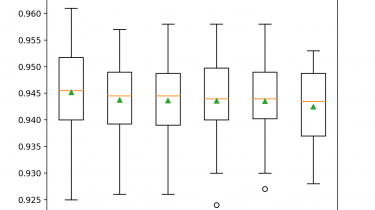# Histogram-Based Gradient Boosting Ensembles in PythonGradient boosting is an ensemble of decision trees algorithms.

It may be one of the most popular techniques for structured (tabular) classification and regression predictive modeling problems given that it performs so well across a wide range of datasets in practice.

A major problem of gradient boosting is that it is slow to train the model. This is particularly a problem when using the model on large datasets with tens of thousands of examples (rows).

Training the trees that are added to the ensemble can be dramatically accelerated by discretizing (binning) the continuous input variables to a few hundred unique values. Gradient boosting ensembles that implement this technique and tailor the training algorithm around input variables under this transform